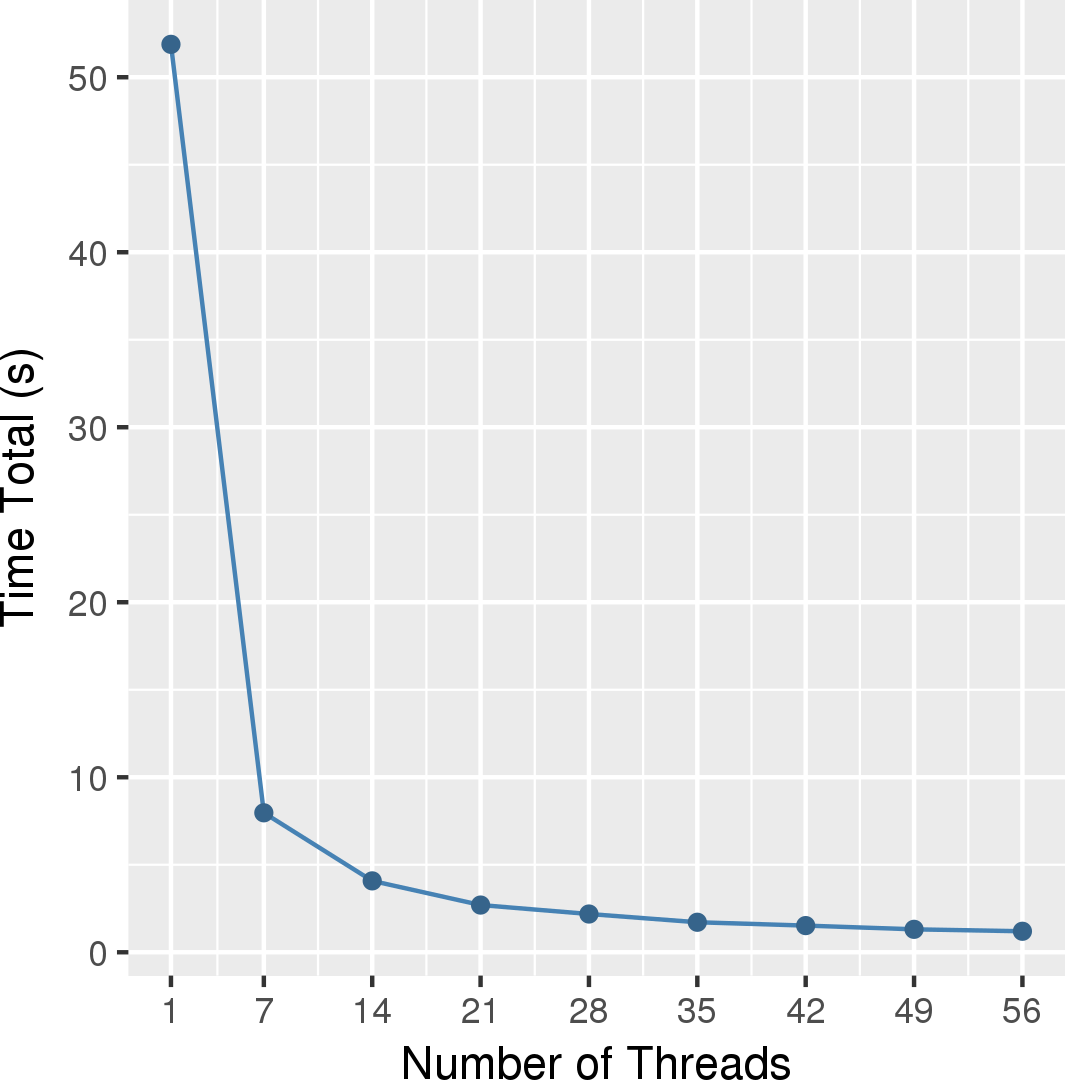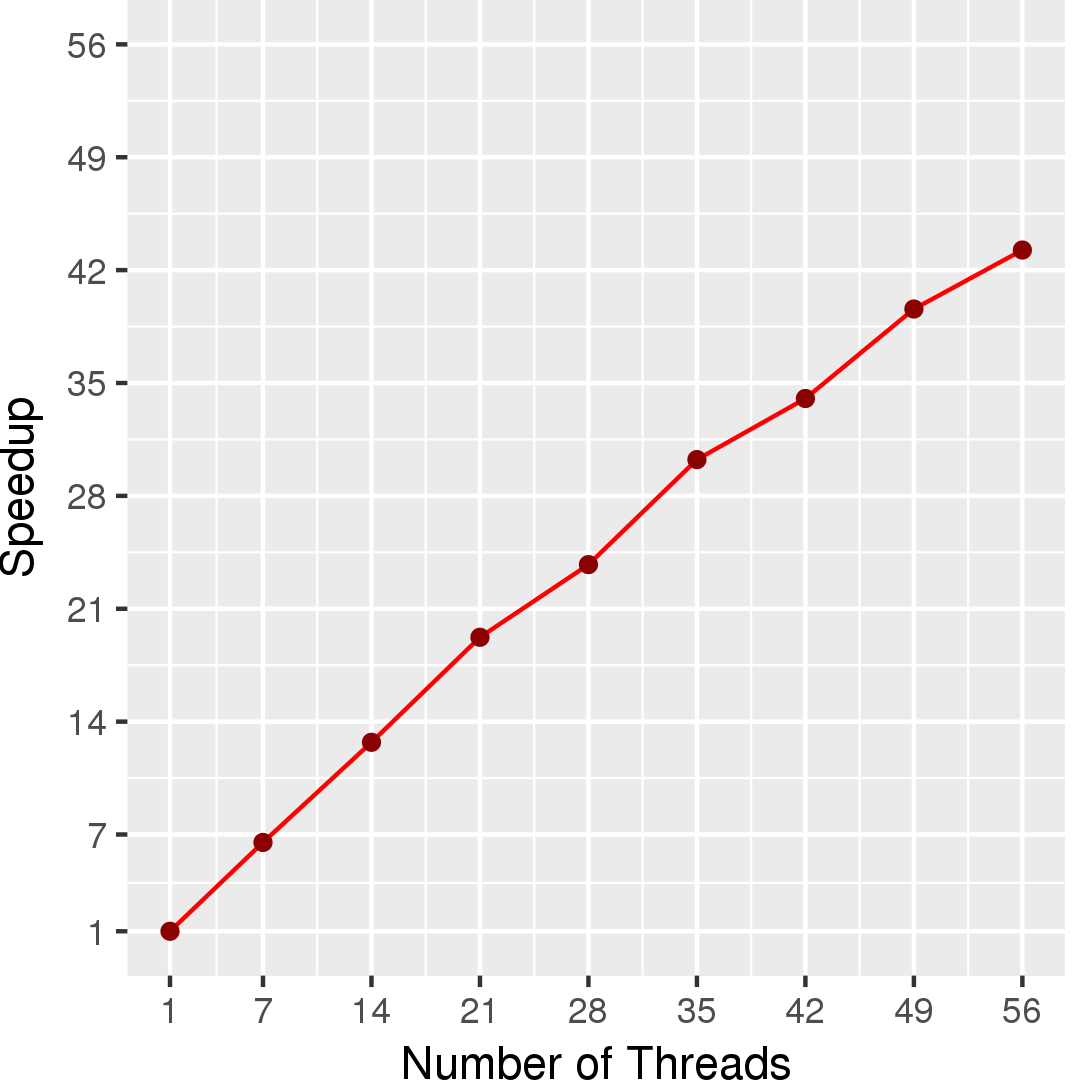# Independent Set

## Application Description

An independent set S of an undirected graph G = (V,E) is a subset of nodes S ⊆ V such that no two nodes share the same edge. Finding the independent set with maximum cardinality |S| is called the maximum independent set problem. Finding such a set in an arbitrary graph is NP-complete.

The problem of finding maximal independent sets is simpler and more useful in practice for large graphs. A maximal independent set is an independent set that cannot be extended with another node and still remain an independent set.

## Algorithm

In the pseudocode below, a node can be in one of the following three states: IN if it is definitely in the independent set, OUT if it is definitely not, and UNDECIDED otherwise.

A simple greedy algorithm solves the maximal independent set problem. Figure 1 gives one implementation.

Initially all nodes are in UNDECIDED state. An UNDECIDED node u is selected from the graph. If none of its neighbors are IN (matched), then the state of u is set to IN and all of its neighbors are set to OUT. This process continues until there are no more UNDECIDED nodes, at which point the nodes in IN state are a maximal independent set.

 1 2 3 4 5 6 7 8 9 10 11 12 Graph g = /* read input */; L1: foreach (Node u : g) { if (u.flag != UNDECIDED) continue; for (Node v : n.getNeighbors()) { if (v.flag == IN) continue L1; u.flag = IN; for (Node v : u.getNeighbors()) v.flag = OUT; } }

Figure 1: Pseudocode for independent set algorithm.

This greedy algorithm requires locks on neighbors which reduce the parallelism and performance. A better way is to give each node a priority. The priority decides whether or not the node is matched among its neighborhood without locks. For more details, please visit http://cs.txstate.edu/~burtscher/research/ECL-MIS/

The Independent Set application implements 6 algorithms:
1. serial: serial greedy version.
2. pull: pull-based greedy version. Node 0 is initially marked IN.
3. detBase: greedy version using Galois deterministic worklist.
4. nondet: greedy version using Galois bulk synchronous worklist.
5. prio(default): based on Martin Butcher's GPU ECL-MIS algorithm.
6. edgetiledprio: edge-tiled version of prio.

Note that Independent Set requires an undirected input graph.

## Data Structures

This application only uses an input undirected graph.

## Performance

Figure 3 and Figure 4 show the execution time in seconds and self-relative speedup (speedup with respect to the single thread performance), respectively, of this algorithm running on a graph generated using rmat model with 2^27 nodes and 2^31 edges.## Machine Description

Performance numbers are collected on a 4 package (14 cores per package) Intel(R) Xeon(R) Gold 5120 CPU machine at 2.20GHz from 1 thread to 56 threads in increments of 7 threads. The machine has 192GB of RAM. The operating system is CentOS Linux release 7.5.1804. All runs of the Galois benchmarks used gcc/g++ 7.2 to compile.